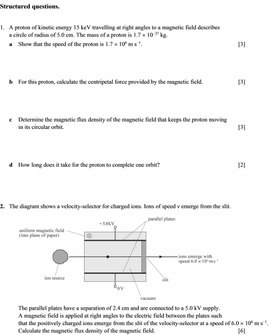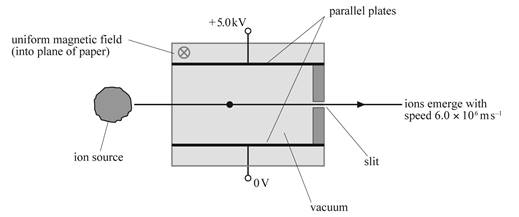9Motion of a charged particle in the magnetic field
Оценка 4.7

# 9Motion of a charged particle in the magnetic field

Оценка 4.7
docx
07.05.20209Motion of a charged particle in the magnetic field.docx

Structured questions.

1.   A proton of kinetic energy 15 keV travelling at right angles to a magnetic field describes
a circle of radius of 5.0 cm. The mass of a proton is 1.7
´ 10−27 kg.

a    Show that the speed of the proton is 1.7 ´ 106 m s−1.                                                           

b    For this proton, calculate the centripetal force provided by the magnetic field.                      

c    Determine the magnetic flux density of the magnetic field that keeps the proton moving
in its circular orbit.                                                                                                            

d    How long does it take for the proton to complete one orbit?                                                 

2.   The diagram shows a velocity-selector for charged ions. Ions of speed v emerge from the slit.The parallel plates have a separation of 2.4 cm and are connected to a 5.0 kV supply.
A magnetic field is applied at right angles to the electric field between the plates such
that the positively charged ions emerge from the slit of the velocity-selector at a speed of 6.0
´ 106 m s−1. Calculate the magnetic flux density of the magnetic field.                                                   

Скачать файл